## Satellite Motion

A satellite is often thought of as being a projectile which is orbiting the Earth. But how can a projectile orbit the Earth? Doesn't a projectile accelerate towards the Earth under the influence of gravity? And as such, wouldn't any projectile ultimately fall towards the Earth and collide with the Earth, thus ceasing its orbit?

These are all good questions and represent stumbling blocks for many students of physics. We will discuss each question here. First, an orbiting satellite is a projectile in the sense that the only force acting upon an orbiting satellite is the force of gravity. Most Earth-orbiting satellites are orbiting at a distance high above the Earth such that their motion is unaffected by forces of air resistance. Indeed, a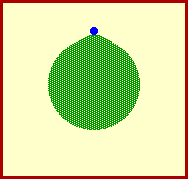satellite is a projectile.

Second, a satellite is acted upon by the force of gravity and this force does accelerate it towards the Earth. In the absence of gravity a satellite would move in a straight line path tangent to the Earth. In the absence of any forces whatsoever, an object in motion (such as a satellite) would continue in motion with the same speed and in the same direction. This is the law of inertia. The force of gravity acts upon a high speed satellite to deviate its trajectory from a straight-line inertial path. Indeed, a satellite is accelerating towards the Earth due to the force of gravity.

Finally, a satellite does fall towards the Earth; only it never falls into the Earth. To understand this concept, we have to remind ourselves of the fact that the Earth is round; that is the Earth curves. In fact, scientists know that on average, the Earth curves approximately 5 meters downward for every 8000 meters along its horizon. If you were to look out horizontally along the horizon of the Earth for 8000 meters, you would observe that the Earth curves downwards below this straight-line path a distance of 5 meters. In order for a satellite to successfully orbit the Earth, it must travel a horizontal distance of 8000 meters before falling a vertical distance of 5 meters. A horizontally launched projectile falls a vertical distance of 5 meters in its first second of motion. To avoid hitting the Earth, an orbiting projectile must be launched with a horizontal speed of 8000 m/s. When launched at this speed, the projectile will fall towards the Earth with a trajectory which matches the curvature of the Earth. As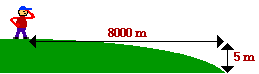such, the projectile will fall around the Earth, always accelerating towards it under the influence of gravity, yet never colliding into it since the Earth is constantly curving at the same rate. Such a projectile is an orbiting satellite.

To further understanding the concept of a projectile orbiting around the Earth, consider the following thought experiment. Suppose that a very powerful cannon was mounted on top of a very tall mountain. Suppose that the mountain was so tall that any object set in motion from the mountaintop would be unaffected by air drag. Suppose that several cannonballs were fired from the cannon at various speeds - say speeds of 8000 m/s, less than 8000 m/s, and more than 8000 m/s. A cannonball launched with speeds less than 8000 m/s would eventually fall to the Earth. A cannonball launched with a speed of 8000 m/s would orbit the Earth in a circular path. Finally, a cannonball launched with a speed greater than 8000 m/s would orbit the Earth in an elliptical path. The animations below depict these ideas.

 Launch Speed less than 8000 m/s Projectile falls to Earth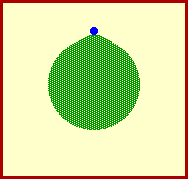Launch Speed less than 8000 m/s Projectile falls to Earth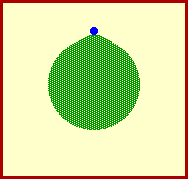Launch Speed equal to 8000 m/s Projectile orbits Earth - Circular Path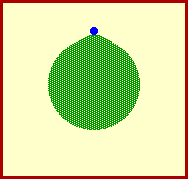Launch Speed greater than 8000 m/s Projectile orbits Earth - Elliptical Path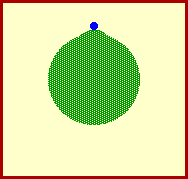Two final notes should be made about satellite motion. First, the 8000 m/s figure used in the above discussion applies to satellites launched from heights just above Earth's surface. Since gravitational influences decrease with the height above the Earth, the orbital speed required for a circular orbit is less than 8000 m/s at significantly greater heights above Earth's surface.

Second, there is an upper limit on the orbital speed of a satellite. If launched with too great of a speed, a projectile will escape Earth's gravitational influences and continue in motion without actually orbiting the Earth. Such a projectile will continue in motion until influenced by the gravitational influences of other celestial bodies.

For more information on physical descriptions of motion, visit The Physics Classroom Tutorial. Detailed information is available there on the following topics:

Newton's Law of Inertia

Acceleration of Gravity

Projectiles

Characteristics of a Projectile's Trajectory